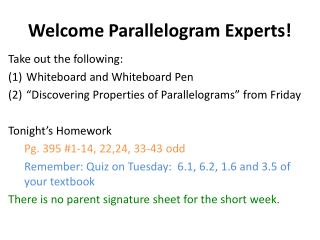DownloadDownload PresentationWelcome Parallelogram Experts!

# Welcome Parallelogram Experts!

Télécharger la présentation## Welcome Parallelogram Experts!

- - - - - - - - - - - - - - - - - - - - - - - - - - - E N D - - - - - - - - - - - - - - - - - - - - - - - - - - -
##### Presentation Transcript

1. Welcome Parallelogram Experts! Take out the following: • Whiteboard and Whiteboard Pen • “Discovering Properties of Parallelograms” from Friday Tonight’s Homework Pg. 395 #1-14, 22,24, 33-43 odd Remember: Quiz on Tuesday: 6.1, 6.2, 1.6 and 3.5 of your textbook There is no parent signature sheet for the short week.

2. Tomorrow’s Quiz Topics: 6.1 – Classifying Polygons / Sum of Interior and Exterior Angles 1.6 – Midpoint and Distance Formula 3.5 – Slope formula 6.2 – Properties of Parallelograms

3. 3 Things

4. Lets’ Warm-Up! On Friday’s Exploration worksheet, write a summary of what you learned after completing this packet. Pretend a first grader wants to know about all the properties of a parallelogram. How would you respond? Be ready to share out!

5. Lets’ Warm-Up! In class on Friday, we discussed #1-3. For #4(c), discuss your answer with someone sitting horizontal to you. Then write your solution on a whiteboard.

6. Lets’ Warm-Up! For #5(e), discuss your answer with someone sitting vertical to you. Then write your solution on a whiteboard.

7. Lets’ Warm-Up! For #6(d), discuss your answer with someone horizontal to you. Then write your solution on a whiteboard.

8. Let’s Warm Up On your whiteboard, draw a parallelogram that includes all necessary tick marks and arch marks.

9. 6-2 Properties of Parallelograms Learning Objective: • SWBAT prove and apply properties of parallelograms to solve problems

10. 6-2 Properties of Parallelograms • Parallelogram • A quadrilateral with two pairs of parallel sides. • To write the name of a parallelogram, you use the symbol .

11. Example/Non-Ex of Parallelograms Example Non-Example

12. 6-2 Properties of Parallelograms What question number does this correlate to on your Exploration Worksheet?

13. 6-2 Properties of Parallelograms On your notes, write down the question number from your Exploration Packet that best matches each theorem.

14. Example 1: Properties of Parallelograms • In CDEF, DE = 74 mm, DG= 31 mm, and mFCD = 42°. Justify each solution. • Find CF. • Find mEFC. • Find CF.

15. Whiteboards In KLMN, LM = 28 in., LN = 26 in., and mLKN= 74°. Justify each response. • Find KN. • Find mNML. • Find LO.

16. Example 2: Using Properties of Parallelograms to Find Measures WXYZis a parallelogram. Find YZ. Find mZ.

17. Whiteboards EFGH is a parallelogram. (a ) Find JG (b) Find FH.

18. Whiteboards • Opposite sides of a parallelogram are ____________ and congruent. • What do the slopes have to be if two lines are parallel to each other?

19. Example 3: Parallelograms in the Coordinate Plane Three vertices of JKLM are J(3, –8), K(–2, 2), and L(2, 6). Find the coordinates of vertex M. Remember: When you are drawing a figure in the coordinate plane, the name gives the order of the vertices.

20. Example 3: Parallelograms in the Coordinate Plane • Step 1: Graph the points. • Step 2: Find the slope of KL by counting the units from K to L. • Step 3: Start at J and count the same number of units. • Step 4: Use the slope formula to verify that

21. Graphing Whiteboard Three vertices of PQRS are P(–3, –2), Q(–1, 4), and S(5, 0). Find the coordinates of vertex R.

22. Math Joke of the Day • What do you call an urgent message sent across a parallel network? • A parallelogram!

23. Example 4: Properties of Parallelograms in a Proof Given: ABCD is a parallelogram. Prove:∆AEB∆CED

24. 3.diags. bisect each other 2.opp. sides  Proof: 1. ABCD is a parallelogram 1. Given 4. SSS Steps 2, 3

25. Whiteboards QRST is a parallelogram. Find each measure. Justify your reasoning. (a) TQ (b) mT

26. Whiteboards In PNWL, NW = 12, PM = 9, and mWLP= 144°. Find each measure (a) PW (b) mPNW

27. Exit Ticket Take out a half sheet of binder paper. Title it: 6.2 Exit Ticket Write your name in the upper right hand corner. • List the key ideas from todays lesson. • Draw and label every side and angle with the given information for PQRS : • PQ= 10 • QR=5 • mS = 110°